# MNIST数据集

MNIST数据集的官网是Yann LeCun’s website。在这里，我们提供了一份python源代码用于自动下载和安装这个数据集。你可以下载这份代码，然后用下面的代码导入到你的项目里面，也可以直接复制粘贴到你的代码文件里面。

import input_data
mnist = input_data.read_data_sets("MNIST_data/", one_hot=True)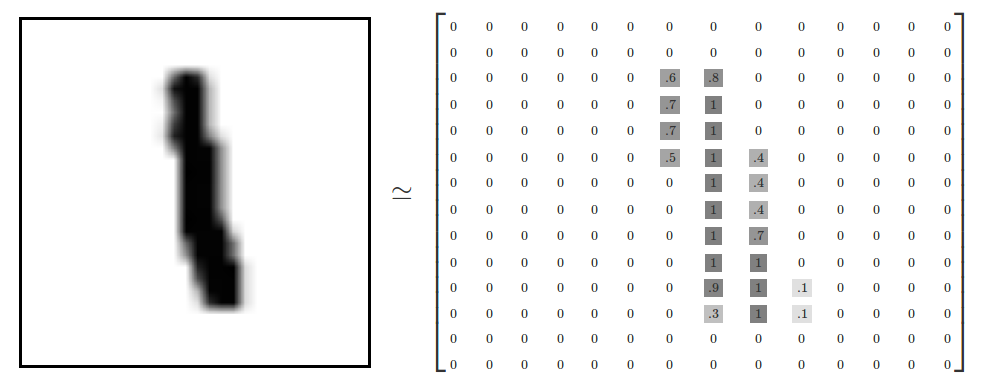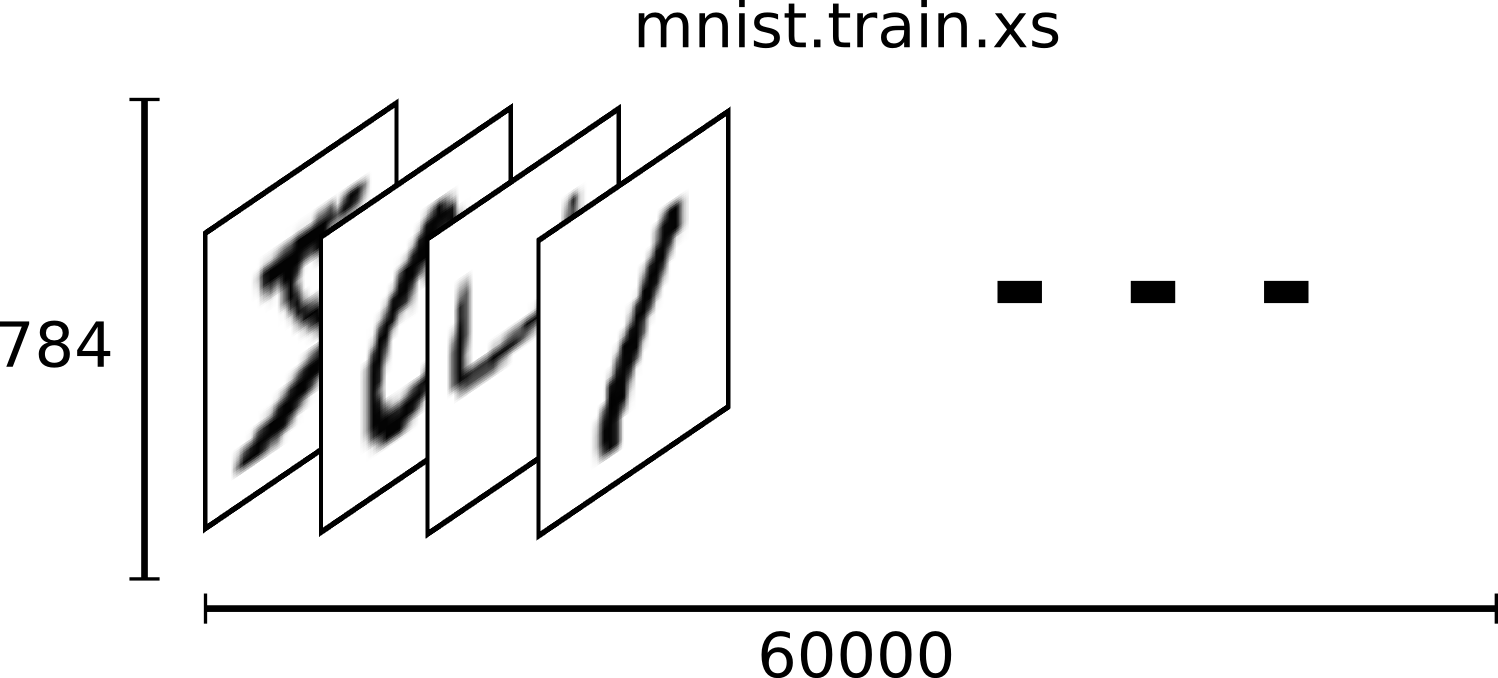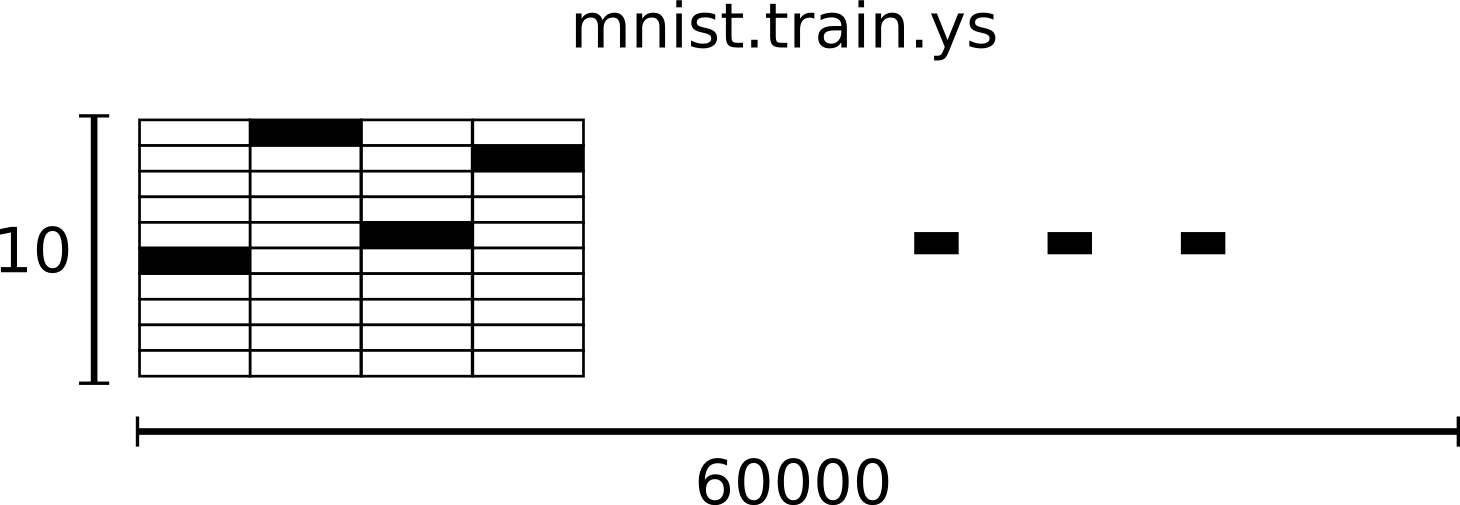# Softmax回归介绍

softmax回归（softmax regression）分两步：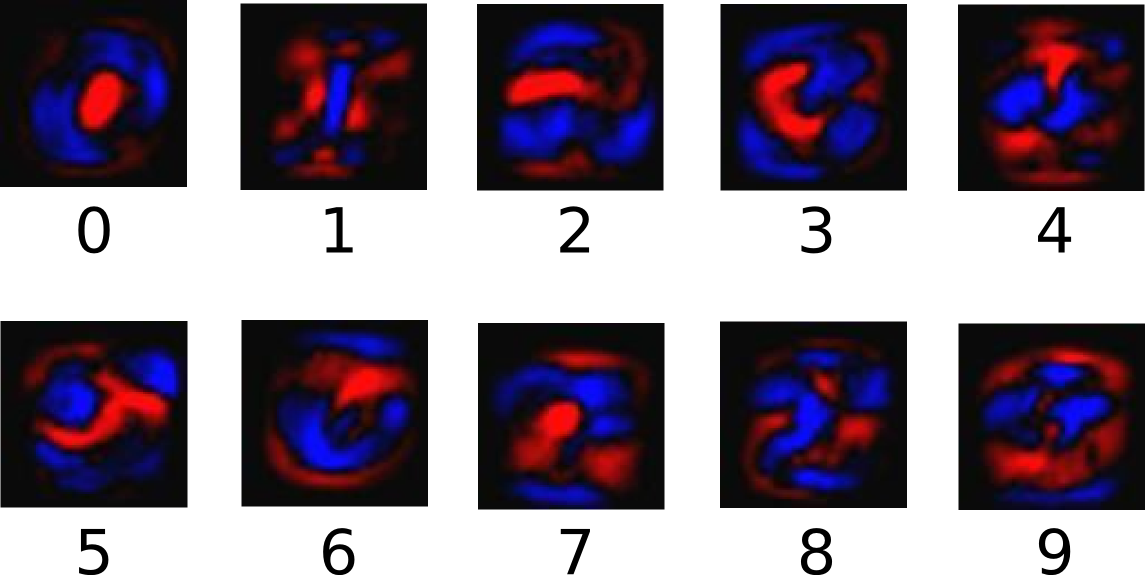evidencei=Wi,jxj+bi

y=softmax(evidence)

softmax(x)=normalize(exp(x))

softmax(x)=exp(xi)jexp(xj)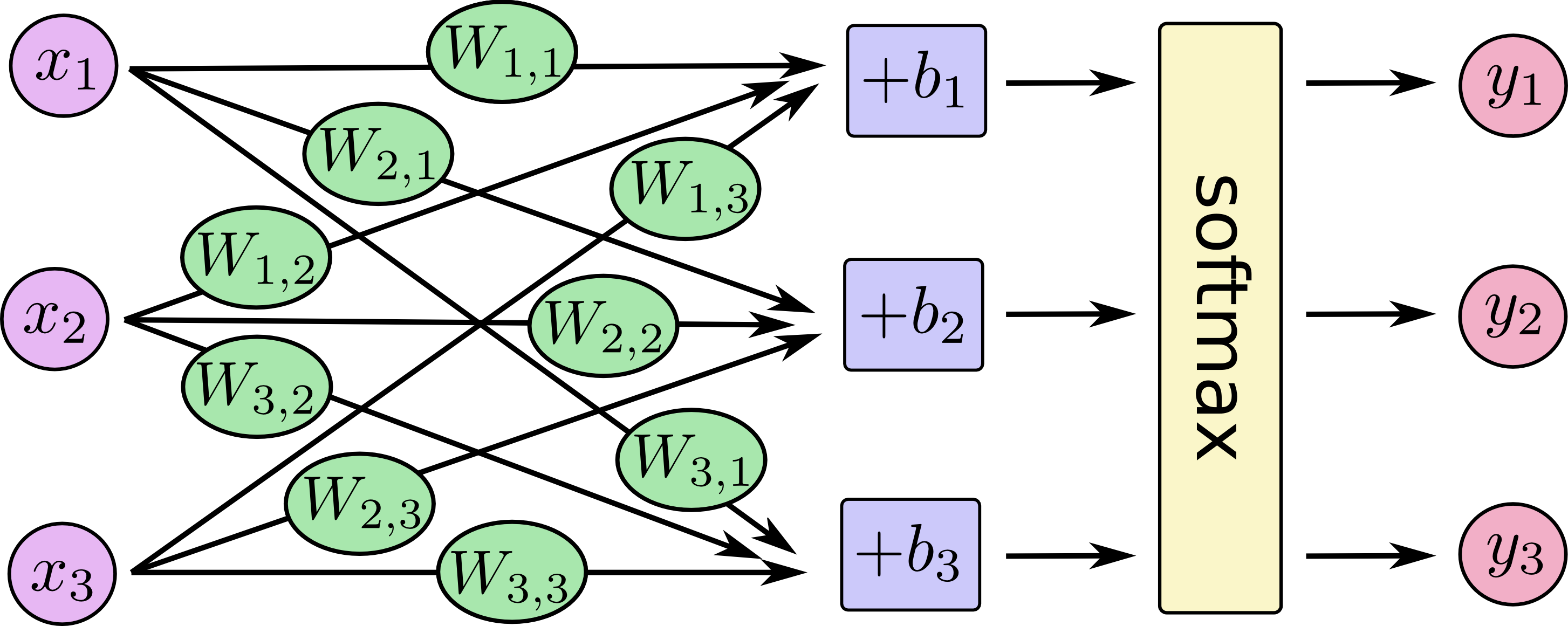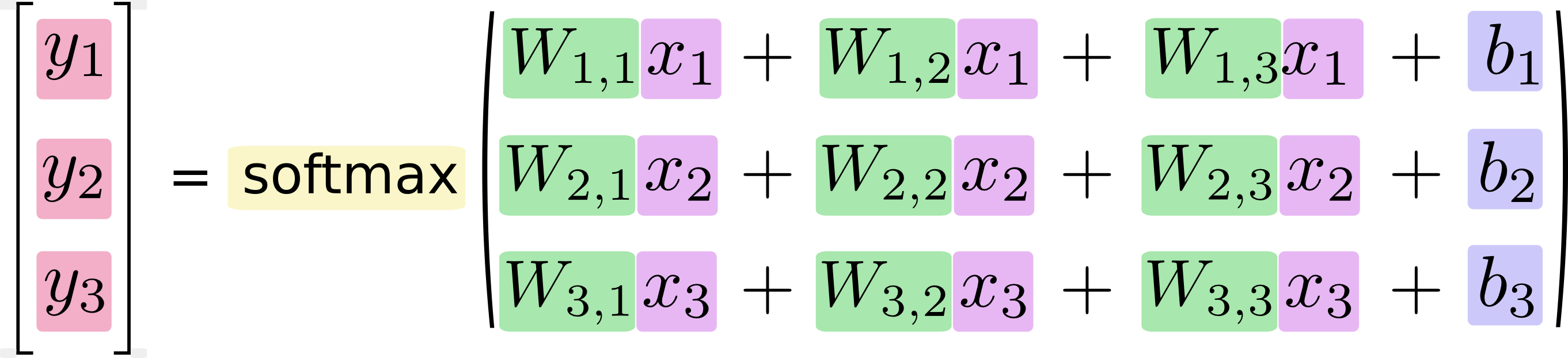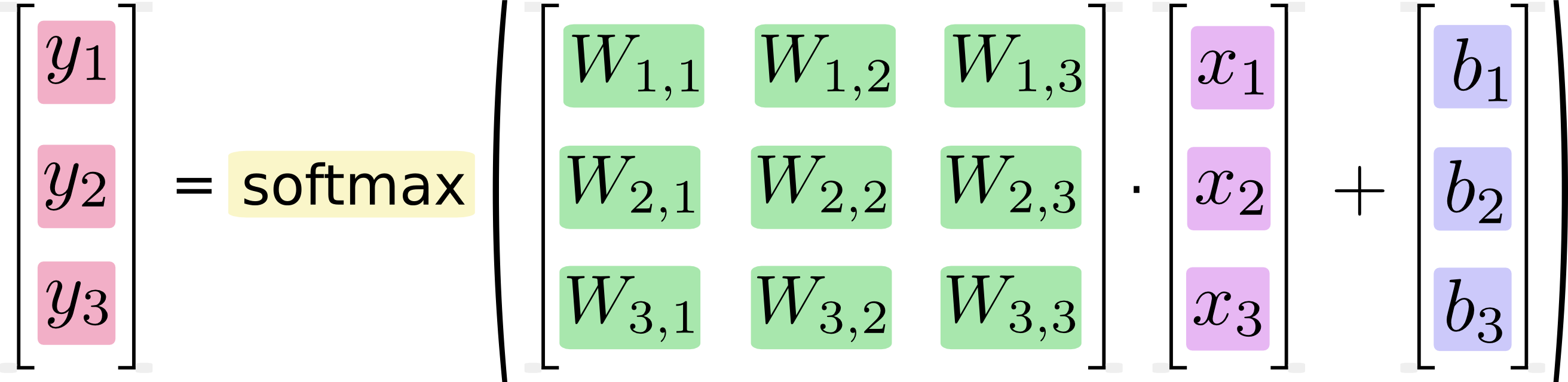y=softmax(Wx)+b

# 实现回归模型

TensorFlow也把复杂的计算放在python之外完成，但是为了避免前面说的那些开销，它做了进一步完善。Tensorflow不单独地运行单一的复杂计算，而是让我们可以先用图描述一系列可交互的计算操作，然后全部一起在Python之外运行。（这样类似的运行方式，可以在不少的机器学习库中看到。）

#coding:utf-8
'''

'''
import tensorflow as tf
import numpy as np
import input_data

#mnist数据输入
mnist = input_data.read_data_sets("MNIST_data/", one_hot = True)

x = tf.placeholder("float", [None, 784]) #placeholder是一个占位符，None表示此张量的第一个维度可以是任何长度的

#
w = tf.Variable(tf.zeros([784,10])) #定义w维度是:[784,10],初始值是0
b = tf.Variable(tf.zeros()) # 定义b维度是:,初始值是0

#
y = tf.nn.softmax(tf.matmul(x,w) + b)

# loss
y_ = tf.placeholder("float", [None, 10])
cross_entropy = -tf.reduce_sum(y_*tf.log(y)) #用 tf.log 计算 y 的每个元素的对数。接下来，我们把 y_ 的每一个元素和 tf.log(y_) 的对应元素相乘。最后，用 tf.reduce_sum 计算张量的所有元素的总和。

# 梯度下降
train_step = tf.train.GradientDescentOptimizer(0.01).minimize(cross_entropy)

# 初始化
init = tf.initialize_all_variables()

# Session
sess = tf.Session()
sess.run(init)

# 迭代
for i in range(1000):
batch_xs, batch_ys = mnist.train.next_batch(100)
sess.run(train_step, feed_dict={x: batch_xs, y_: batch_ys})
if i % 50 == 0:
correct_prediction = tf.equal(tf.argmax(y, 1), tf.argmax(y_, 1))
accuracy = tf.reduce_mean(tf.cast(correct_prediction, "float"))
print "Setp: ", i, "Accuracy: ",sess.run(accuracy, feed_dict={x: mnist.test.images, y_: mnist.test.labels})

# 评估模型
#correct_prediction = tf.equal(tf.argmax(y, 1), tf.argmax(y_, 1))
#accuracy = tf.reduce_mean(tf.cast(correct_prediction, "float"))
#print sess.run(accuracy, feed_dict={x: mnist.test.images, y_: mnist.test.labels})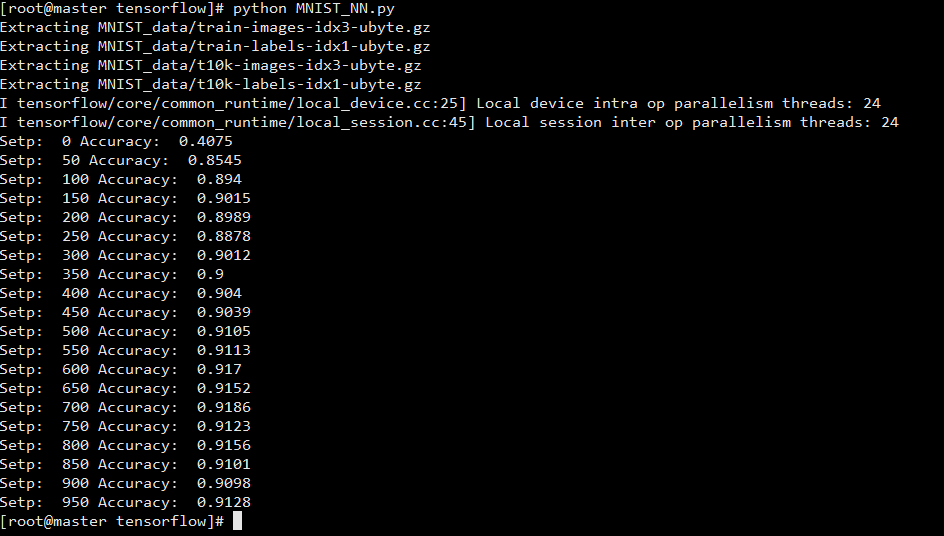# 参考

[tensorflow中文社区]（http://tensorfly.cn/tfdoc/tutorials/mnist_beginners.html